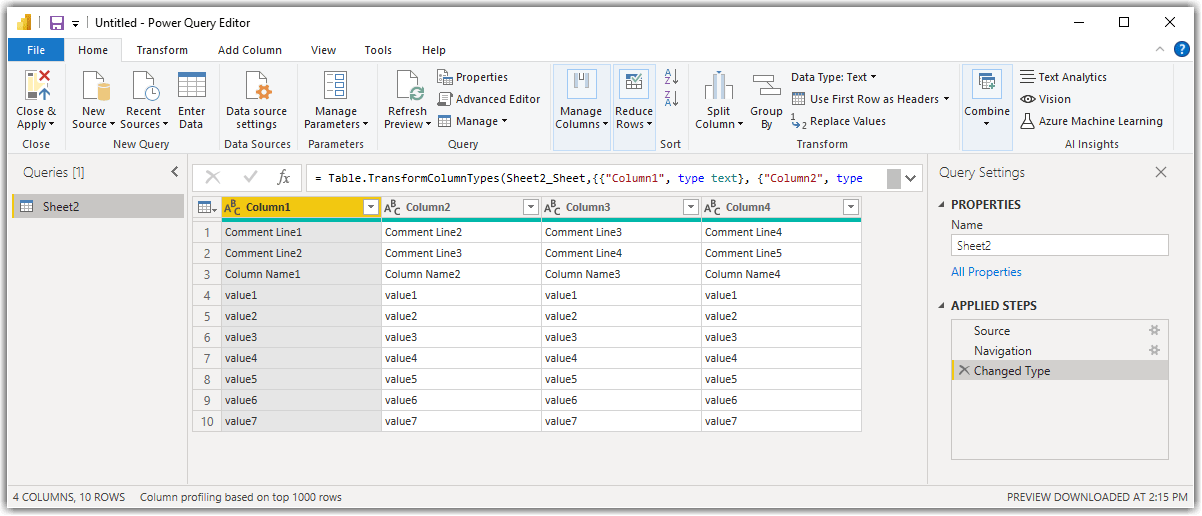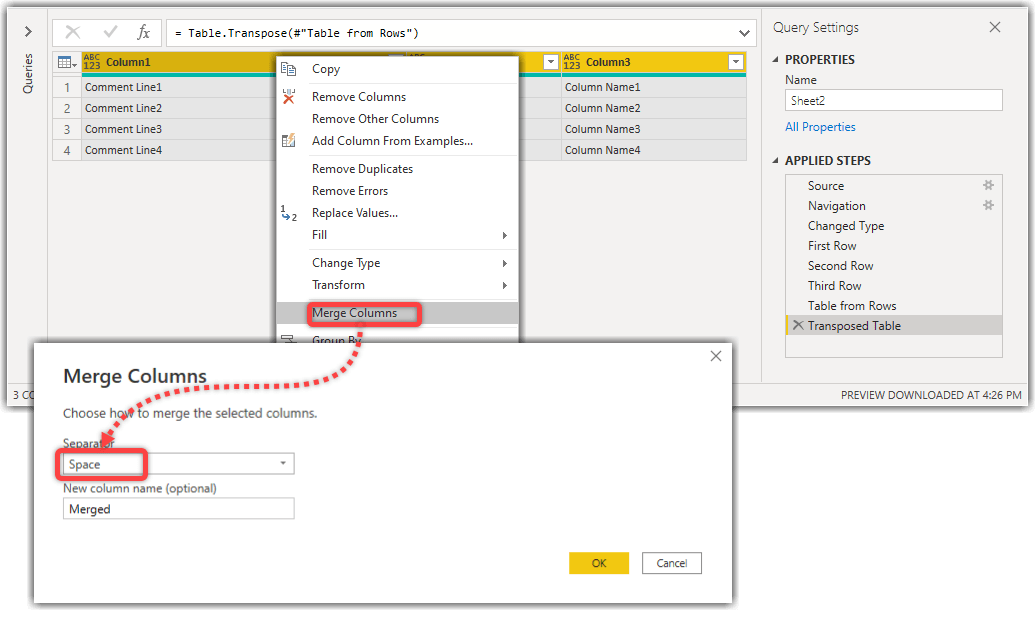# Combining X Number of Rows in Power Query for Power BI, Excel and Power Query Online

A while back, I was working on a project involving getting data from Excel files. The Excel files contain the data in sheets and tables. Getting the data from the tables is easy. However, the data in the sheets have some commentaries on top of the sheet, then the column names and then the data itself. Something like below:

This approach is pretty consistent across many Excel files. The customer wants to have the commentary in the column names when the data is imported into Power BI. So the final result must look like this:

The business requirement though is to combine the first 3 rows of data and promote it as the column name.

## The Challenge

Let’s connect the Excel file and look at the data in Power BI Desktop.

As you can see in the preceding image, Power BI, or more precisely, Power Query, sees the data in Table format. After we click the Transform Data button, this is what we get in Power Query Editor:Connected to sample data from Power Query in Power BI Desktop

We all know that tables consist of Columns and Rows. The conjunction of a column and a row is a Cell. What we require to do is to concatenate the values of cells from the first three rows. We also have to use a Space character to separate the values of each cell from the others.

In Power Query, we can get each row of data in as a Record with the following syntax:

``Table{RecordIndex}``

In the above syntax, the `Table` can be the results of the previous transformation step, and the `RecordIndex` starts from `0`. So to get the first row of the table in the preceding image, we use the following syntax:

``#"Changed Type"{0}``

Where the ` #"Changed Type" `is the previous step. Here are the results of running the preceding expression:

So we can get the second and third rows with similar expressions. The following image shows the entire codes in the Advanced Editor:

But how do we concatenate the values of the rows?

My approach is:

• Creating a table from the first three rows
• Transposing the resutls to get the rows as columns
• Merging the columns into a single column
• Transposing the new column
• Appending the transposed values to the original table
• Promoting the first row as column names

Here is how we do so:

1. We create a Table from Records using the Table.FromRecords() function:
1. Transposing the resutls to get the rows as columns
1. Merging all columns and removing the columns. To do so we select all columns, right-click a selected column and click Merge Columns. We use Space as the separatorMerging columns as a new column in Power Query
1. Transposing the new merged column
1. Appending the transposed results to the original table. The resutls of the third transformation step gives us the orignial table. We use the `Table.Combine()` function
``Table.Combine({#"Transposed Table1", #"Changed Type"})``

As you can in the preceding image, the results are not what we want as we still have the first 3 rows appearing in the values. So before we append the transposed results to the original table, we have to remove the first 3 rows from the original table, and then append the results. To remove the first N rows we use `Table.Skip()` function. So we have to change the previous step as follows:

``Table.Combine({#"Transposed Table1", Table.Skip(#"Changed Type", 3)})``Appending the transposed results to the results of removing the first 3 rows of the original data
1. Promoting the first row as column names

Easy!

Hmm… Not really. Especially when we have multiple other Excel files; some have two, some have three rows of comments, and some have even more. So not only is the task time-consuming, but it also is pretty boring and prone to human errors. What if there is a custom function that I can invoke to get the job done?

## The Solution

To overcome the challenge, I wrote a pretty dynamic custom function. It accepts a table and an iterator. The iterator is the number of rows we want to concatenate and use as the column names. After invoking the function, it iterated through the first X number of rows following the scenario I explained in the previous section. It then appends the transposed results to the original table while removing the first X number of rows.

Here you go…

When I tested my original function with different data types, I noted that I had to convert the comment values to text data type; otherwise, dynamically, the merging columns step breaks as it cannot concatenate text and number values.

A big shout-out to Imke Feldman for writing such helpful blogposts. I used her technique in one block of the following code to dynamically convert the columns to text.

``````// fn_CombineTopXRows
(InputTable as table, Iterator as number) as table =>
let
TopXRows = List.Generate(
() => 0
, each _ < Iterator
, each _ + 1
, each InputTable{_}
),
TableFromRecords = Table.FromRecords(
List.Generate(
() => 0
, each _ < Iterator
, each _ + 1
, each InputTable{_}
)
),
TransposeTable = Table.Transpose(
TableFromRecords
, List.Generate(
() => 0
, each _ < Iterator
, each _ + 1
, each "Col" & Text.From(_)
)
),

// Special thanks to Imke Feldman for this line of code?
// https://www.thebiccountant.com/2017/01/09/dynamic-bulk-type-transformation-in-power-query-power-bi-and-m/
DataTypeToText = Table.TransformColumnTypes(
TransposeTable
, List.Transform(
Table.ColumnNames(TransposeTable)
, each {_, type text}
)
),

CombineColumns = Table.CombineColumns(
DataTypeToText
, List.Generate(
() => 0
, each _ < Iterator
, each _ + 1
, each "Col" & Text.From(_)
)
, Combiner.CombineTextByDelimiter(
" "
, QuoteStyle.None
)
,"Merged"
),
TransposeCombined = Table.Transpose(CombineColumns),
AppendToOriginalTable = Table.Combine({TransposeCombined, Table.Skip(InputTable, Iterator)}),
in

You can download the code from here.

Let’s see the `fn_CombineTopXRows` custom function in action. Here are the results after invoking the function with our previous sample data:

Here is a more extreme sample data containing special characters, nulls and Unicode characters:

And here are the results of invoking the `fn_CombineTopXRows `custom function:

As always, if you have any comments or you know a better way than I explained here, please let me know via the comments section below.

## Update

After sharing this post on LinkedIn, Muneer Marzouq, commented that he would write the custom function differently. I liked his approach, so I share his code here.

``````// fn_CombineTopNRows

(InputTable as table, TopNRows as number, optional Delimiter as nullable text) as table =>
let
Delimiter = if Delimiter <> null then Delimiter else "#(lf)",
ToTables = Table.Split(InputTable, TopNRows),
ColsNames = Table.ColumnNames(ToTables{0}),
ToText =
Table.TransformColumnTypes(
ToTables{0}
, List.Transform(
ColsNames
, each {_, type text}
)
),
ToColumns = Table.ToColumns(ToText),
CombineText =
List.Transform(
ToColumns
, each Text.Combine(_
, Delimiter
)
),
ToTable = Table.FromRows({CombineText}, ColsNames),
Combine = Table.Combine({ToTable, ToTables{1}})
in
Combine``````

## Update 2022 Jan

Here is another solution that Mahmoud Baniasadi put in the comments section. What I like about Mahmoud’s code are:

• Using the `Table.SplitAt()` function which returns a list of two tables instead of `Table.Split()` function which returns a list of tables
• Implementing the function documentation
``````let func=
(InputTable as table, TopNRows as number, optional Delimiter as nullable text) as table =>
let

Delimiter = if Delimiter <> null then Delimiter else "#(lf)",
// Correction: Change Table.Split to Table.SplitAt
ToTables = Table.SplitAt(InputTable, TopNRows),
ColsNames=Table.ColumnNames(ToTables{0}),
ToText= Table.TransformColumnTypes(
ToTables{0}
, List.Transform(
ColsNames
, each {_, type text}
)
),

/* My alternative method for this part
CombineText=
List.Transform(
Table.ToList(
Table.Transpose(ToText),
Combiner.CombineTextByDelimiter(Delimiter,0)),
each Text.Trim(_)),
*/

// Muneer Marzouq Solution
ToColumns=Table.ToColumns(ToText),
CombineText =
List.Transform(
ToColumns
, each Text.Combine(_
, Delimiter
)
),
//End of Muneer code which is different from mine

ToTable = Table.FromRows({CombineText}, ColsNames),
Combine = Table.Combine({ToTable, ToTables{1}}),
// Correction: add one step to promote combined rows as header
in
documentation = [
Documentation.Name = " fn_CombineTopNRows ",
Documentation.Description = " Returns a Table with Combining X Number of Rows. ",
Documentation.LongDescription = " Returns a Table with Combining X Number of Rows and Promote them as header. ",
Documentation.Category = " Table ",
Documentation.Source = " https://www.biinsight.com/combining-x-number-of-rows-in-power-query-for-power-bi-excel-and-power-query-online/ ",
Documentation.Version = " 1.0 ",
Documentation.Author = " Muneer Marzouq, Mahmoud Bani Asadi, Soheil Bakhshi ",
Documentation.Examples = {[Description = " see this blogpost: https://www.biinsight.com/combining-x-number-of-rows-in-power-query-for-power-bi-excel-and-power-query-online/ ",
Code = " fn_CombineTopNRows(#table({""Column1"", ""Column2"", ""Column3""}, {{""TitleA"", ""TitleC"", ""Title1""}, {""TitleB"", ""TitleD"", ""Title2""}, {7, 8, 9}}), 2, "" "")",
Result = " #table({""TitleA TitleB"", ""TitleC TitleD"", ""Title1 Title2""}, {{7, 8, 9}}) "]}]
in

This is why I love knowledge sharing. You learn something new from others every day.

## 2 thoughts on “Combining X Number of Rows in Power Query for Power BI, Excel and Power Query Online”

1.Mahmoud Bani Asadi says:

Thanks for great topic and all solutions:

As an update of Mr. Marzouq’s suggestion I correct some missing and show part of it which is different from what I did (as a comment) and add documentation.

let func=
(InputTable as table, TopNRows as number, optional Delimiter as nullable text) as table =>
let

Delimiter = if Delimiter <> null then Delimiter else “#(lf)”,
// Correction: Change Table.Split to Table.SplitAt
ToTables = Table.SplitAt(InputTable, TopNRows),
ColsNames=Table.ColumnNames(ToTables{0}),
ToText= Table.TransformColumnTypes(
ToTables{0}
, List.Transform(
ColsNames
, each {_, type text}
)
),

/* My alternative method for this part
CombineText=
List.Transform(
Table.ToList(
Table.Transpose(ToText),
Combiner.CombineTextByDelimiter(Delimiter,0)),
each Text.Trim(_)),
*/

// Muneer Marzouq Solution
ToColumns=Table.ToColumns(ToText),
CombineText =
List.Transform(
ToColumns
, each Text.Combine(_
, Delimiter
)
),
//End of Muneer code which is different from mine

ToTable = Table.FromRows({CombineText}, ColsNames),
Combine = Table.Combine({ToTable, ToTables{1}}),
// Correction: add one step to promote combined rows as header
in
documentation = [
Documentation.Name = ” fn_CombineTopNRows “,
Documentation.Description = ” Returns a `Table` with Combining X Number of Rows. “,
Documentation.LongDescription = ” Returns a `Table` with Combining X Number of Rows and Promote them as header. “,
Documentation.Category = ” Table “,
Documentation.Source = ” https://www.biinsight.com/combining-x-number-of-rows-in-power-query-for-power-bi-excel-and-power-query-online/ “,
Documentation.Version = ” 1.0 “,
Documentation.Author = ” Muneer Marzouq, Mahmoud Bani Asadi, Soheil Bakhshi “,
Documentation.Examples = {[Description = ” see this blogpost: https://www.biinsight.com/combining-x-number-of-rows-in-power-query-for-power-bi-excel-and-power-query-online/ “,
Code = ” fn_CombineTopNRows(#table({“”Column1″”, “”Column2″”, “”Column3″”}, {{“”TitleA””, “”TitleC””, “”Title1″”}, {“”TitleB””, “”TitleD””, “”Title2″”}, {7, 8, 9}}), 2, “” “”)”,
Result = ” #table({“”TitleA TitleB””, “”TitleC TitleD””, “”Title1 Title2″”}, {{7, 8, 9}}) “]}]
in
2.Soheil Bakhshi says: